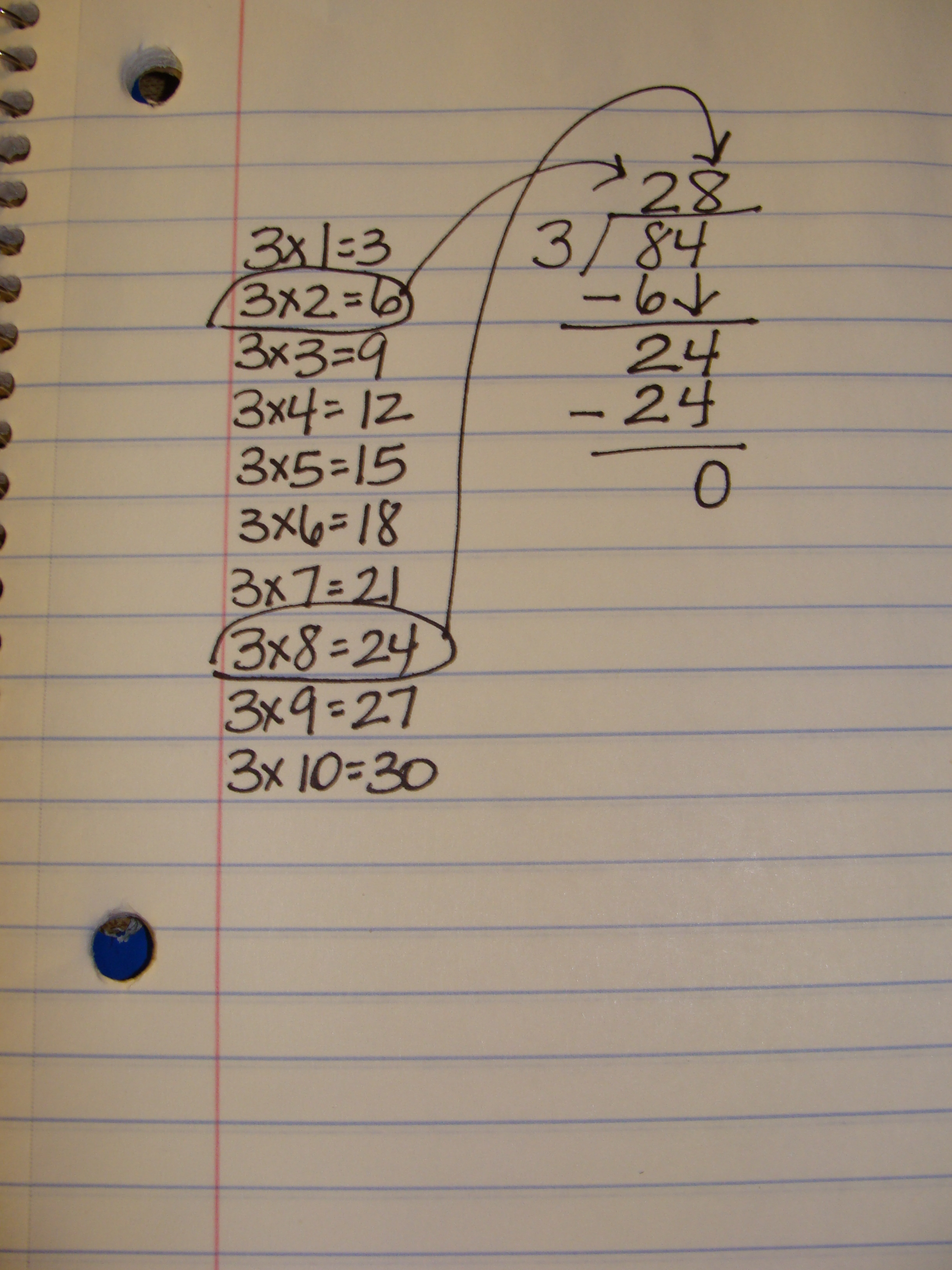Great Math Products!

# How to Effectively Teach Long Division with One Digit Divisors

A struggle for many students when learning long division is their lack of fluency with multiplication facts. To help students adapt to learning the process of long division, allow them to write their multiples using the divisor in the margin of their paper.  This is one less mental process students must go through when solving a long division problem.  I have seen this strategy prove effective with students who are emerging in their long division.  For an example, when students are solving 84 divided by 3, they can write all of the multiples of 3 down the margin of their papers as a cheat sheet of sorts.If students use the traditional algorithm, they can immediately look down the list and find that 3×2=6 and 3×3 =9.  Since the first digit of 84 is 8, students can look at their list and find that 3×2=6 yields the largest number that will fit into 8 and that 3×3=9 is too large.  When subtracting the 6 tens from 84, the difference is 24.  Students can look down the multiples list again to see that 3×8 =24 to find the ones place of the divisor.  This method of teaching division can be found in Marilyn Burns’ book Extending Division.